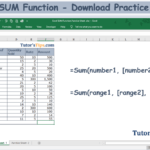The Excel SUM Formula is used, where we need to do the sum of the numerical values in the selected range, cells reference or table column/row. It can sum together the numerical values in the multiple selected ranges or cells references.

## The feature of “SUM” function: –

• With the help of the SUM Function, we can get the total of the numerical values in the selected array, range or cells.
• It can use further with other excel function.
• Error values or text values that cannot be recognized into numbers are not summed by this function.
• The logical values TRUE and FALSE are also not summed.
• Empty cells and text are also not summed.

### Explanation of Function:-

Now, We will explain the Arguments of the Function.

=SUM(number1, [number2], [number3], …)

number1: – Number 1 is represented as the first selected cell which we want to sum up.

[number2]: – Number 2 is represented the next selected cell which we want to sum up.

[number3]: – Number 3 is represented the next selected cell which we want to sum up and a process of selection going on.

We can also select range instead of selecting a number individually.

=SUM(range1, [range2], [range3], …)

range1: – Range 1 is represented as the first selected cells which we want to sum up.

[range2]: – Range 2 is represented the next selected cells which we want to sum up.

[range3]: – Range 3 is represented the next selected cells which we want to sum up and a process of selection going on.

### Example of Function: –

I will show you, how to apply this function.

From the following table get the total of the amount.

Solution:-

We will Apply the SUM Formula and get the result. This is shown in the following steps: –

Step No. 1:-

We will write the “=SUM( ” in the column of the result.

Step No. 2

Now select the range of cells on which you want to apply the function.

Step No. 3: –

Now, Press enter and get the result: –

Thanks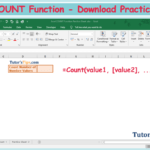The Excel COUNT Function is used, where we need to count the number of numbers in the selected range or cells reference. It can count together the numerical values in the multiple selected ranges or cells references.

• With the help of COUNT Function, we can get the total number of the number values in the selected array, range or cells.
• It can use further with other excel function.
• Error values or text values that cannot be recognized into numbers are not counted by this function.
• The logical values TRUE and FALSE are also not counted.
• Empty cells and text are also not counted.

### Explanation of Function:-

Now, We will explain the Arguments of the Function.

=COUNT(value1, [value2], …)

value1: – Value 1 is represented the selected range of the cells from which the function have to count the number of numbers values.

[value2]: – Value 2 is represented the second selected range of the cells from which the function have to count the number of numbers values. Because we can use multiple selections. If you have only single row or column to select then you don’t need to enter the second value.

### Example of Function: –

I will show you, how to apply the function.

From the following table get the total of number values in the values column.

Solution:-

We will Apply the COUNT function and get the result. This is shown in the following steps: –

Step No. 1:-

We will write the “=COUNT( ” in the column of the result.

Step No. 2

Now select the range of cells on which you want to apply the function.

Step No. 3: –

Now, Press enter and get the result: –

Thanks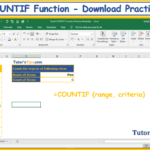## Excel COUNTIF Function: –

The Excel COUNTIF Function is used where we need to count the numbers of repeated values on single criteria. It can count the value of the cells based on a condition of any Text, number and logical text. It is also working on matching concepts like VLOOKUP and HLOOKUP but with a condition.

### The feature of “COUNTIF” function: –

• With the help of COUNTIF Function, we can retrieve the data if the condition is matched with the selected cells.
• It helps in applying a particular condition on the data.

### The logical operator: –

The “COUNTIF” function we can also use follow logical test on the selected data other than the Text and Number.

 Logical Test Meaning = Equal to <> Not Equal to > Greater than >= Greater than and Equal to < Less than <= Less than and equal to

### Explanation of Function:-

Now, We will explain the Arguments of the Function.

=COUNTIF (range, criteria)

range: – Range is that column or row or both of selected cells on which we want to apply the condition or criteria.

criteria: –  This is a condition which we have to apply to the selected cells.

### Example of Function: –

I will show you, how to apply the whole function. (using optional also).

From the following table get the item-wise value of the total sale amount.

Solution:-

We will Apply the COUNTIF function and get the result. This is shown in the following steps: –

Step No. 1:-

We will write the “=COUNTIF( ” in the column of the result.

Step No. 2

Now select the cells on which you want to apply the Condition or criteria.

Step No. 3

Now select the Condition or criteria which you want to apply.

Step No. 4: –

Now, Press enter and get the result: –

Thanks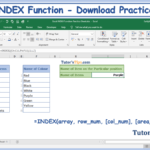## Excel Index Function:-

The Excel Index function is used to find out the value in a selected array or range by giving a position of the cell in the selected array. We will direct the excel to find out the value of cells on the particular position of the cell. This position can be provided dynamically by the match formula. I will explain it further in the next topics.

### The feature of “Index” function: –

• With the help of Index Function, we can retrieve the value of the cell in the selected array by providing the position of the cell.
• It helps in getting a particular value of the certain position of the cell in the selected array.
• We can provide position dynamically by the match function.

### Explanation of Function:-

Now, We will explain the Arguments of the Function.

`=INDEX(array, row_num, [col_num], [area_num])`

array: –

An array is that area from which we will get the value of the cell by providing the position of the cell.

row_num: –

The row_num that selected row number or dynamically provided the row number by match function to the excel to get the value of the cell from the selected array.

col_num: –

The row_num that selected Column number or dynamically provided the column number by match function to the excel to get the value of the cell from the selected array.

area_num:-

When we have selected the two or more array then we will provide the number of the array.

### Example of Function: –

I will show you, how to apply the whole function. (using optional also).

From the following table get the value of the selected position of the cell: –

Solution:-

We will Apply the Match function and get the result. This is shown in the following steps: –

Step No. 1:-

We will write the “=Index( ” in the column of the result.

Step No. 2

Now select the cells from which you want to get the value of the particular position.

We can select multiple arrays in single index formula. Like shown in the following:-

Step No. 3

Now select the position of the value in the row.

Step No. 4

Now select the position of the value in the Column. If multiple columns selected then we need it rather then its default value is equal to 1.

Step No. 5

Now select the number of the array, if we have selected multiple arrays like shown in the example.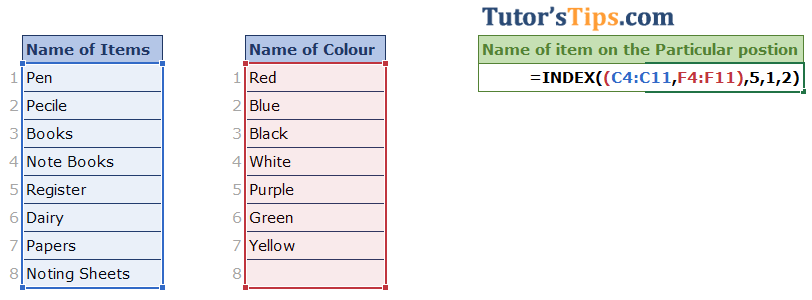After all these steps we will get the result shown as follow: –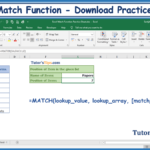## Excel Match Function:-

The Excel Match function is used to find out the position of the selected value in the selected array. The position of the selected value may be exact matched, less than and more than from the value.

### The feature of “Match” function: –

• With the help of Match Function, we can retrieve the position of the Selected value in the selected array and we can use this position further with index function and others also.
• It helps in getting a particular position of a certain value in the selected array.

### Explanation of Function:-

Now, We will explain the Arguments of the Function.

`=MATCH(lookup_value, lookup_array, [match_type])`

lookup_value: –

The lookup_value that value of which we want to know the position in the selected array.

lookup_array: –

The lookup_array that selected cells from which we want to know the position of the lookup_value.

match_type: –

The match_type are of three types show as following: –

1.  If we put the value “1”  – The MATCH function will find the largest value that is less than or equal to value. You should be sure to sort your array in ascending order.
2. If we put the value “0”  –  The MATCH function will find the first value that is equal to value. The array can be sorted in any order.
3. If we put the value “-1” – The MATCH function will find the smallest value that is greater than or equal to value. You should be sure to sort your array in descending order.

### Example of Function: –

I will show you, how to apply the whole function. (using optional also).

From the following table get the position of the papers in the selected array: –

Solution:-

We will Apply the Match function and get the result. This is shown in the following steps: –

Step No. 1:-

We will write the “=Match( ” in the column of the result.

Step No. 2

Now select the cells with which you want to match the value with the selected array.

Step No. 3

Now select the array.

Step No. 4

Now apply matching condition

Step No. 5: –

Now, Copy and paste the function in all remaining cell to get the result.

Thanks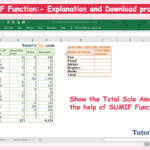The Excel SUMIF Function is used where we need to sum up the value on single criteria. It can be sum up the value of the cells based on a condition of any Text, number and logical text. It is also working on matching concepts like VLOOKUP and HLOOKUP but with a condition.

### The feature of “SUMIF” function: –

• With the help of SUMIF Function, we can retrieve the data if the condition is matched with the selected cells date.
• It helps in applying a particular condition on the data.

### The logical operator: –

The “SUMIF” function we can also use follow logical test on the selected data other than the Text and Number.

 Logical Test Meaning = Equal to <> Not Equal to > Greater than >= Greater than and Equal to < Less than <= Less than and equal to

### Explanation of Function:-

Now, We will explain the Arguments of the Function.

=SUMIF(range, criteria, [sum_range])

range: – Range is that column or row of selected cells on which we want to apply the condition or criteria.

criteria: –  This is a condition which we have to apply to the selected cells.

[sum_range]: – Sum range is that range of selected cells, which consist of the values which we want to total or summed up. This is optional because in the case where the range and sum_ range are the same. It means if we want to make a total of the value when a condition is cleared or matched from the other range of cells, not from the same column or row of the selected range on.

### Example of Function: –

I will show you, how to apply the whole function. (using optional also).

From the following table get the item-wise value of the total sale amount.

Solution:-

We will Apply the SUMif function and get the result. This is shown in the following steps: –

Step No. 1:-

We will write the “=SUMIF( ” in the column of the result.

Step No. 2

Now select the cells on which you want to apply the Condition or criteria.

In an example, we have to sum up the sale amount as per the name of the item. So, we have to apply a condition to the name of the items.

Step No. 3

Now select the Condition or criteria which we want to apply.

In an example, The name of items are given, we have to write the total amount in front of them, so we can select the criteria by selecting the cell of the given name.

Step No. 4

Now select the range of the cells of which the value we have to sum up.

In an example, The total amount of sale date wise give in the “F” column, so we select the cells of “F” column which have amount of sales.

Step No. 5: –

Now, Copy and paste the function in all remaining cell to get the result.

Thanks

## IF Function in Excel – Explanation with example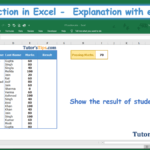## IF Function in Excel: –

The “IF” Function in Excel is a logical test, which represents the result in two way if the logical condition true and false. There is always two way of getting the value, one is when we got our condition/logic true and another we will get when the condition/logic false. We can use this formula where we want to apply the condition of less than, greater than and equal to. It means if the value of the selected cell is less than from specific amount then answer the specific true value(it may be in numeric or in words) rather then answer the false value.

### The feature of “IF” function: –

• With the help of IF Function, we can retrieve the data if the condition is cleared by the data.
• VLOOKUP and HLOOKUP shows only matched value but “IF” function shows the value in both ways.
• It helps in applying a particular condition on the data.

### The logical operator: –

The “IF” function, We can use only to follow a logical test on the selected data only.

 Logical Test Meaning = Equal to <> Not Equal to > Greater than >= Greater than and Equal to < Less than <= Less than and equal to

### Explanation of Function:-

Now, We will explain the Arguments of the Function.

=IF(logical_test, [value_if_true], [value_if_false])

logical_test:- The Logical test is the value or condition which will provide us with the result of the selected cell as true or false. It is a specific condition/test as explained in the above table.

value_if_true: – The value if true is that value which you want to show if the condition or logical test is true.

value_if_false: – The value if false is that value which you want to show if the condition or logical test is false.

### Example of Function: –

In the following table, we want to retrieve the result of all students in Pass and Fail Form. The minimum passing marks are 70.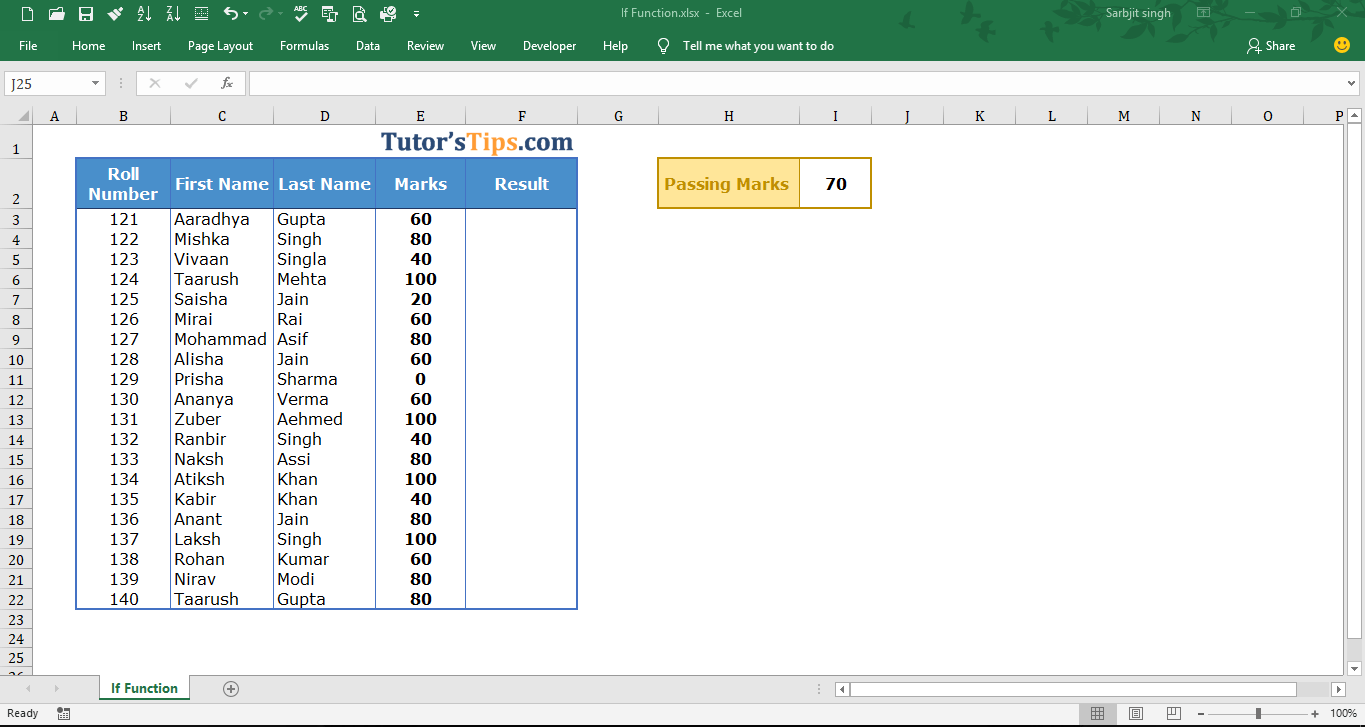Solution:-

We will Apply the if function and get the result. This is shown in the following steps: –

Step No. 1:-

We will write the “=if( ” in the column of the result.

Step No. 2

Now select the cell on which you want to apply the logical test. and then write the logical test

Or if can by selecting the cell where the value of logic is written and then fix this cell by adding “\$” signs.

Step No. 3: –

Now, write the answer which you want if the condition show when the condition is true. Write the answer in t invited comma.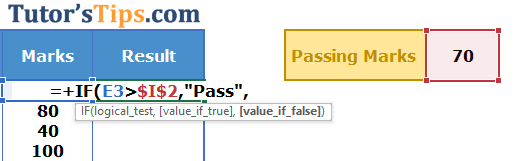Example of If – Solution Step no. 3

Step No. 4: –

Now, write the answer which you want if the condition show when the condition is false. Write the answer in t invited comma.

Step No. 5: –

Now, Copy and paste the function in all remaining cell to get the result.

Thanks

## HLOOKUP in Excel – Explanation with example## HLOOKUP in Excel:

In the HLOOKUP Function “H” stand for horizontal and with the help of this formula, we can retrieve the matched value from the selected cells(table array) from up to down means in horizontal order. It supports approximate and exact matched value. It can only look up and retrieve the values in the horizontal order. The lookup value must be in the first row in the selected cells(table array).

### Need for HLOOKUP formula: –

• With the help of HLOOKUP, we can retrieve the numbers of exact or approximate matched value in the seconds.
• It helps in saving time and doing work more efficiently.
• it will show the matched value in the column/row where you want. So, we don’t need to find the values individually from the selected date and then copy, paste it.

### The Feature of HLOOKUP formula: –

1. It works only up to down. It can only look up the value from the Selected cell(Table_array) from up to the downside. it means from the rows.
2. This formula provides two types of matched value i.e. approximate and exact.
3. HLOOKUP always finds the first matched value from the selected cell(Table_array) and retrieve it.
4. We can use 1 and 0 instead of Ture and False respectively.
5. It retrieves data based on the Row number.

### Explanation of Formula:-

Now, We will explain the Arguments of the formula.

=HLOOKUP(lookup_value, table_array, row_index_num, [range_lookup])

lookup_value: –  The lookup value is that value, which will find the exact matched value from the selected cells with this formula. This value is always written in the first row of the selected cells(table array) because HLOOKUP only finds the value in the horizontal order from the selected cells(table array).

table_array: – This is the array of selected cells from which the HLOOKUP will find and retrieve the matched value.

row_index_num: – It means Row Index Number. The number of that row from which we want to retrieve the matched value. The number of rows is counted from the first row of the selected cells(table array).

range_lookup: – It means what type of match you want to show. There is two types of match shown as following: –

1. Ture: – Approximate Match
2. False: – Exact Match

If you want to retrieve the Exact match value to show, then write the false and if you approximate matched value, then write true.

### Example of Formula: –

In the following table, we want to retrieve the predetermined percentage of increment from the table according to the appraisal score.

Solution:-

We will use the VLOOKUP formula to retrieve the predetermine the percentage of increment according to the appraisal score.

1st Step: –

We will write the “=HLOOKUP( ” in the column of the percentage of increment.

2nd Step: –

Select the value which we common in both table i.e. Appraisal Score and add a comma at the end.

3rd Step: –

Select the Table array from which we want to retrieve the value and add a comma at the end.

4th Step: –

Fix the selection of table array by adding the dollar sign (\$) in both the starting and end of the selection like shown below: –

This step is needed where we did not want to update the selection of the table array when we copy the whole formula in the remaining cell.

5th Step: –

Now select the number of the column of the selected table array of which the value you want to retrieve.

6th Step:

Now choose the mode of matching if you want to match exact value then enter false or 0 or if you want to match approximate value then enter true or 1.

Now, press enter key, the formula is complete and you will get the result.

Step No. 7: –

So copy this result(means formula) and then paste it in the remaining cell in which you want to use the same.

Thanks

## VLOOKUP in Excel – Explanation with example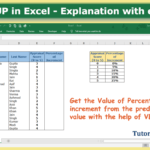## What is VLOOKUP: –

In VLOOKUP, V stands for vertical and with the help of this formula, we can retrieve the matched value from the selected cells(table array) from left to right means in vertical order. It supports approximate and exact matched value. It can only look up and retrieve the values in the vertical order. The lookup value must be in the first column in the selected cells(table array).

### Need for VLOOKUP formula: –

• With the help of VLOOKUP, we can retrieve the numbers of exact or approximate matched value in the seconds.
• It helps in saving time and doing work more efficiently.
• it will show the matched value in the column/row where you want. So, we do not need to find the values individually from the selected date and then copy, paste it.

### The Feature of VLOOKUP formula: –

1. It works only left to right. It can only look up the value from the Selected cell(Table_array) from left to right side.
2. This formula provides two types of matched value i.e. approximate and exact.
3. VLOOKUP always finds the first matched value from the selected cell(Table_array) and retrieve it.
4. We can use 1 and 0 instead of True and False respectively.
5. It retrieves data based on column number.

### Explanation of Formula:-

Now, We will explain the Arguments of the formula.

=VLOOKUP(lookup_value, table_array, col_index_num, [range_lookup])

lookup_value: –  The lookup value is that value, which will find the exact matched value from the selected cells(table array) with this formula. This value is always written in the first column of the selected cells(table array) because VLOOKUP only finds the value in the vertical order from the selected cells.

table_array: – This is the array of selected cells from which the VLOOKUP will find and retrieve the matched value.

col_index_num: – It means Column Index Number. The number of that column from which we want to retrieve the matched value. The number of columns is counted from the first column of the selected cells(table array).

range_lookup: – It means what type of match you want to show. There are two types of match shown as following: –

1. Ture: – Approximate Match
2. False: – Exact Match

If you want to retrieve the Exact match value to show the write the false and if you approximate match then write true.

Example of Formula: –

In the following table, we want to retrieve the predetermined percentage of increment from the table according to the appraisal score.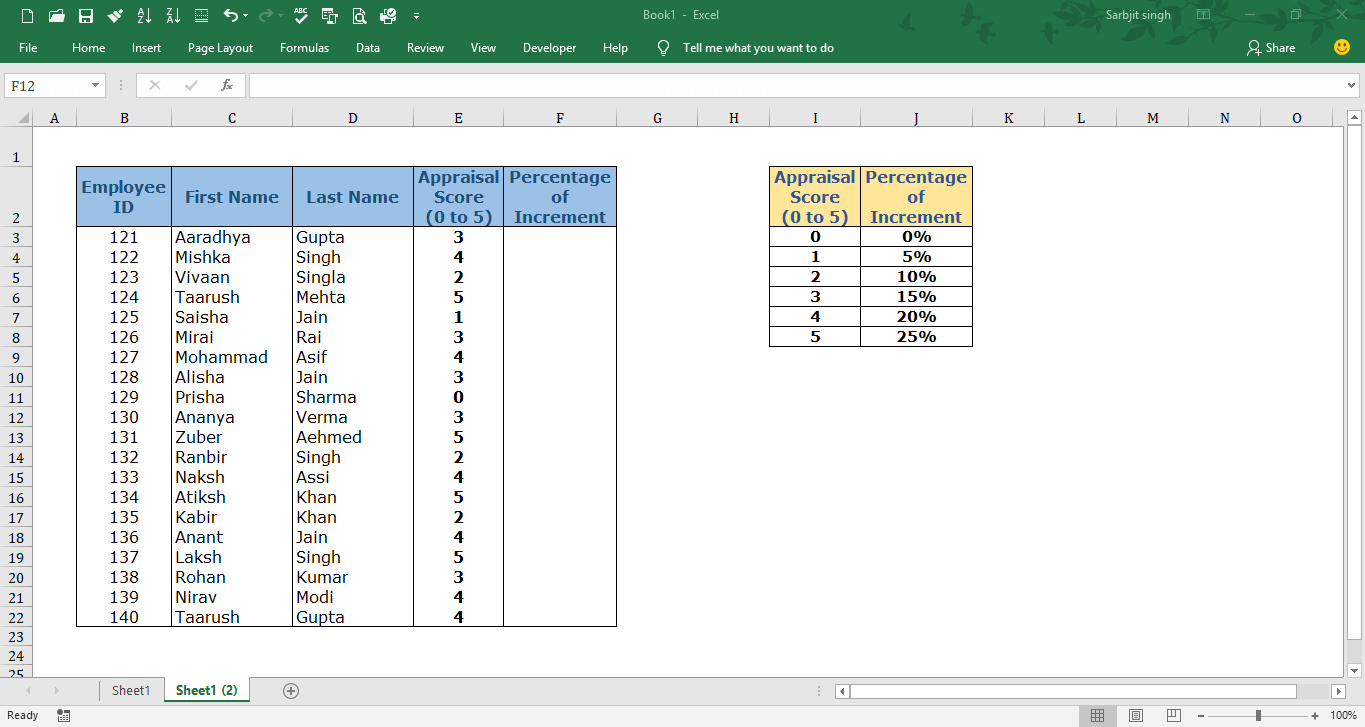Solution:-

We will use the VLOOKUP formula to retrieve the predetermine the percentage of increment according to the appraisal score.

1st Step: –

We will write the “=VLOOKUP( ” in the column of the percentage of increment.

2nd Step: –

Select the value which we common in both table i.e. Appraisal Score and add a comma at the end.

=VLOOKUP(E3,

3rd Step: –

Select the Table array from which we want to retrieve the value and add a comma at the end.

4th Step: –

Fix the selection of table array by adding the dollar sign (\$) in both the starting and end of the selection like shown below: –

This step is needed where we did not want to update the selection of the table array when we copy the whole formula in the remaining cell.

5th Step: –

Now select the number of the column of the selected table array of which the value you want to retrieve.

6th Step:

Now choose the mode of matching if you want to match exact value then enter false or 0 or if you want to match approximate value then enter true or 1.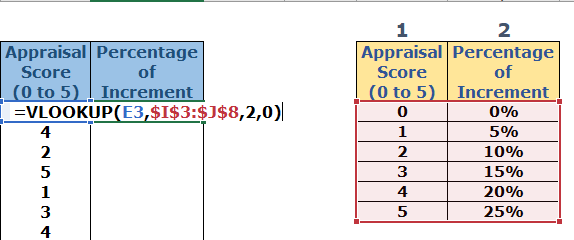Now, the formula is complete and you will get the result.

Step No. 7: –

So copy this result(means formula) and then paste it in the remaining cell in which you want to use the same.